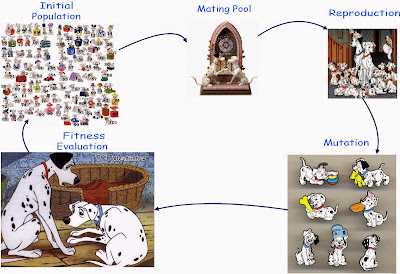## Sunday, 22 December 2013

### Genetic ProgrammingA Typical GP Breeding Cycle
Genetic programming (GP) is another very important machine learning (ML) algorithm. It is also a very elegant algorithm and possibly the most powerful too. It belongs to the class of evolutionary algorithms (EAs). To this end, it is also a coarse adaptation of Darwinian evolution and tries to manifest the themes of natural selection and the survival of fittest in problem solving as they are  perceived about natural  biological systems.

In traditional ML algorithms the search space is comprised of all the possible solutions in terms of the possible values for the various coefficients for a known or a priori fixed mathematical model. The  underlying mathematical model may have been chosen to solve any real world problem. For instance, the mathematical model may be required for weather forecasting, financial bidding or robotic control of a machine. A traditional ML algorithm is run on such a model so as to tune its coefficients so that it starts performing optimally in some meaningful sense.

GP differs from traditional ML algorithms that simply aim at tuning the coefficients of a well known model. In GP the search space is comprised of all the possible computer programs or mathematical models that may be candidate solutions of an underlying problem. To this end, while other ML algorithms search the so called coefficient space for possible solutions of a problem, GP tries to find an optimal solution from the whole program space, whatever that may be.

Running GP is quite simple and there are loads of open source software that are available online. A typical breeding cycle of GP is also quite similar to a typical EA. Following is the pseudo code for a typical breeding cycle of GP. It has been taken from a discourse on genetic algorithms

1. Create an initial population of individuals of size n.
2. Evaluate each individual on the given problem and assign it a rank R.
3. Randomly choose pairs of individuals as some function of R.
4. Apply genetic operators of crossover and mutation on the chosen individuals to form a pair of offspring.
5. Repeat step 4 until a new population is formed.
6. Go to step 2 and keep repeating till this point until the desired solution is found.